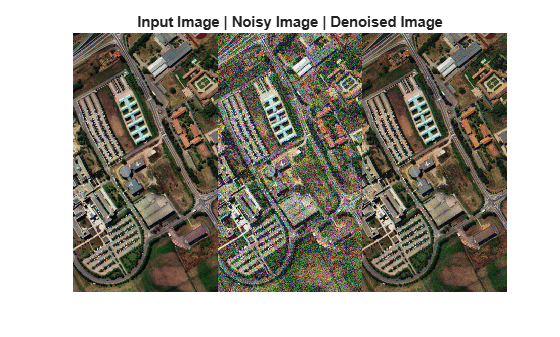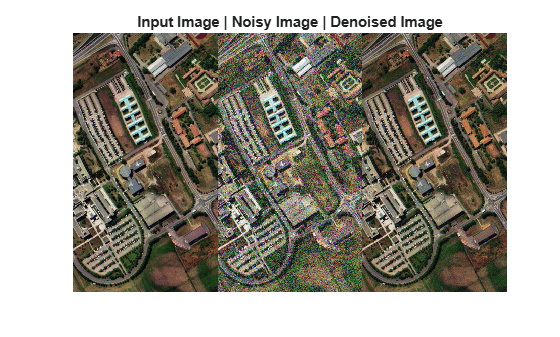# denoiseNGMeet

Denoise hyperspectral images using non-local meets global approach

## Syntax

``outputData = denoiseNGMeet(inputData)``
``outputData = denoiseNGMeet(inputData,Name,Value)``

## Description

example

````outputData = denoiseNGMeet(inputData)` reduces noise in hyperspectral data by using the non-local meets global (NGMeet) approach. This is an iterative approach that integrates both the spatial non-local similarity and spectral low-rank approximation for estimating the original pixel values. For more information, see Algorithms.```

example

````outputData = denoiseNGMeet(inputData,Name,Value)` also specifies options using one or more name-value pair arguments. Use this syntax to set the parameter values for NGMeet approach. NoteThis function requires the Image Processing Toolbox™ Hyperspectral Imaging Library. You can install the Image Processing Toolbox Hyperspectral Imaging Library from Add-On Explorer. For more information about installing add-ons, see Get and Manage Add-Ons. ```

## Examples

collapse all

Read hyperspectral data into the workspace.

`hcube = hypercube('paviaU.hdr');`

Normalize the input data and add Gaussian noise to the normalized input data.

```hcube = hypercube(rescale(hcube.DataCube),hcube.Wavelength); inputData = imnoise(hcube.DataCube,'Gaussian',0,0.005); inputData = assignData(hcube,':',':',':',inputData);```

Denoise the noisy hyperspectral data using NGmeet method.

`outputData = denoiseNGMeet(inputData);`

Estimate RGB images for the input, noisy, and the denoised output datacube. Increase the image contrast by applying contrast stretching.

```originalImg = colorize(hcube,'Method','rgb','ContrastStretching',true); noisyImg = colorize(inputData,'Method','rgb','ContrastStretching',true); denoisedImg = colorize(outputData,'Method','rgb','ContrastStretching',true);```

Display RGB images of the original, noisy, and denoised data.

```figure montage({originalImg,noisyImg,denoisedImg}) title('Input Image | Noisy Image | Denoised Image');```Read hyperspectral data into the workspace.

`hcube = hypercube('paviaU.hdr');`

Normalize the input data and add Gaussian noise to the normalized input data.

```hcube = hypercube(rescale(hcube.DataCube),hcube.Wavelength); inputData = imnoise(hcube.DataCube,'Gaussian',0,0.005); inputData = assignData(hcube,':',':',':',inputData);```

Denoise the noisy hyperspectral data by using the NGmeet method. Set the smoothing parameter value to 0.01 and the number of iterations to 4.

`outputData = denoiseNGMeet(inputData,'Sigma',0.01,'NumIterations',4);`

Estimate RGB images for the input, noisy, and the denoised output datacube. Increase the image contrast by applying contrast stretching.

```originalImg = colorize(hcube,'Method','rgb','ContrastStretching',true); noisyImg = colorize(inputData,'Method','rgb','ContrastStretching',true); denoisedImg = colorize(outputData,'Method','rgb','ContrastStretching',true);```

Display RGB images of the original, noisy, and denoised data.

```figure montage({originalImg,noisyImg,denoisedImg}) title('Input Image | Noisy Image | Denoised Image');```## Input Arguments

collapse all

Input hyperspectral data, specified as a 3-D numeric array that represent the hyperspectral data cube of size M-by-N-by-C or `hypercube` object. If the input is a `hypercube` object, the function reads the data cube stored in the `DataCube` property of the object. The hyperspectral data cube must be real and non-sparse.

### Name-Value Arguments

Specify optional comma-separated pairs of `Name,Value` arguments. `Name` is the argument name and `Value` is the corresponding value. `Name` must appear inside quotes. You can specify several name and value pair arguments in any order as `Name1,Value1,...,NameN,ValueN`.

Example: `denoiseNGMeet(hcube,'Sigma',0.3)`

Smoothing parameter, specified as the comma-separated pair consisting of `'Sigma'` and a positive scalar. The default value is 0.1 times the noise variance (σn). Increasing this value, increases the level of smoothing in the denoised output.

Data Types: `single` | `double` | `int8` | `int16` | `int32` | `int64` | `uint8` | `uint16` | `uint32` | `uint64`

Number of spectral bands in low-rank approximation, specified as the comma-separated pair consisting of `'SpectralSubspace'` and a positive integer scalar in the range (0, C]. C is the number of bands in the input data. The number of endmembers in the hyperspectral data can be a good estimate for the number of spectral bands to use for low-rank approximation. You can find the number of endmembers in the input hyperspectral data by using the `countEndmembersHFC` function.

Data Types: `single` | `double` | `int8` | `int16` | `int32` | `int64` | `uint8` | `uint16` | `uint32` | `uint64`

Number of iterations, specified as the comma-separated pair consisting of `'NumIterations'` and a positive integer scalar. Increase this value for better denoising results.

Data Types: `single` | `double` | `int8` | `int16` | `int32` | `int64` | `uint8` | `uint16` | `uint32` | `uint64`

## Output Arguments

collapse all

Denoised hyperspectral data, returned as a 3-D numeric array or `hypercube` object.

## Algorithms

The NGMeet method estimates the denoised data cube by using these steps. For each iteration, i

1. Compute spectral low-rank approximation of the noisy input data (Yi) by using singular value decomposition. The approximation results in a reduced data cube (Mi) and the related orthogonal basis Ai.

2. Perform spatial denoising of the reduced data cube Mi by using non-local similarity filtering. You can control the degree of smoothing by specifying the smoothing parameter `'Sigma'`.

3. Perform inverse projection. Map the denoised reduced data cube Mi to original space by using the orthogonal basis Ai. The result is the denoised output (Xi) obtained at iteration i.

4. Perform iterative regularization. Update the noisy input data, Yi+1 = λXi + (1-λ)Yi.

5. Repeat steps 1 to 4, for the specified number of iterations. The final value Xi is the denoised hyperspectral data.

 He, Wei, Quanming Yao, Chao Li, Naoto Yokoya, and Qibin Zhao. “Non-Local Meets Global: An Integrated Paradigm for Hyperspectral Denoising.” In 2019 IEEE/CVF Conference on Computer Vision and Pattern Recognition (CVPR), 6861–70. Long Beach, CA, USA: IEEE, 2019. https://doi.org/10.1109/CVPR.2019.00703.